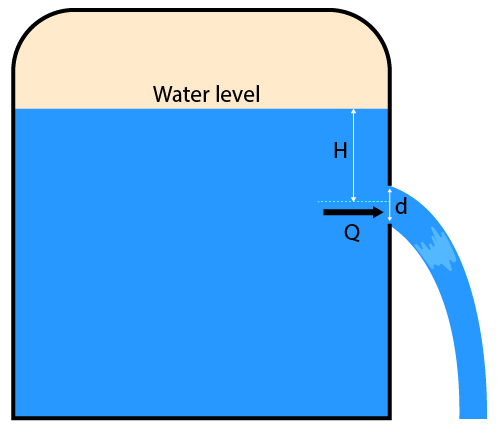# Coefficient of Discharge Calculator

Created by Krishna Nelaturu
Last updated: Sep 05, 2022

Our coefficient of discharge calculator can help you determine how different the actual discharge rate is compared to the theoretical flow rate. It can also tell you the resistance against the flow of the fluid. If you're keen on learning more about the discharge coefficient and flow resistance, join us in a brief discussion about the fundamentals of the discharge coefficient below.

## What is coefficient of discharge?

The actual discharge rate in a hydraulic system tends to be different from the discharge rate we calculate on paper due to certain irrevocable losses (such as friction loss). The coefficient of discharge is the ratio between these two discharge rates. Mathematically, we can write:

$C_d = \frac{Q_\text{act}}{Q_\text{th}}$

Where:

• $C_d$ - Coefficient of discharge;
• $Q_\text{act}$ - Actual discharge rate; and
• $Q_\text{th}$ - Theoretical discharge rate.

The coefficient of discharge is a dimensionless parameter. It is one of the three such hydraulic coefficients:

• Coefficient of discharge $C_d$;
• Coefficient of contraction $C_c$; and
• Coefficient of velocity $C_v$.

The relationship between these three dimensionless parameters is:

$C_d = C_c \cdot C_v$

The discharge coefficient reflects the resistance against the fluid flow through the hydraulic system. Measured as the flow resistance $k$, it is given by the reciprocal of the square of the discharge coefficient:

$k = \frac{1}{C_d^2}$

## Formula for coefficient of discharge

We can measure the discharge at an orifice using mass flow rate $\text{(in kg/s)}$ or volumetric flow rate $\text{(in m}^3\text{/s)}$.

The theoretical discharge rate through an orifice is given by:

$\small Q_\text{th} \text{(in kg/s)} = \rho A c = A \sqrt{2\rho\Delta P} \\ Q_\text{th} \text{(in m}^3\text{/s)} = A \sqrt{2gH}$

Where:

• $\rho$ - Fluid density;
• $A$ - Cross section of the orifice;
• $c$ - Flow velocity;
• $\Delta P$ - Change in pressure through the orifice;
• $g$ - Acceleration due to gravity; and
• $H$ - Fluid head or the height of the fluid surface from the orifice's center.

Combining this with its definition, we can derive a formula for the coefficient of discharge at an orifice:

$\small C_d = \frac{Q_\text{act}}{Q_\text{th}} = \frac{Q_\text{act}\text{(in kg/s)}}{A \sqrt{2\rho\Delta P}}\\[1em] C_d = \frac{Q_\text{act}\text{(in m}^3\text{/s)}}{A \sqrt{2gH}}$

## How to calculate coefficient of discharge

To use the formula of coefficient of discharge, we must either know the fluid density and the change in pressure or the fluid head. In both cases, it is vital to know the cross-sectional area and the actual flow rate. We can measure this actual discharge rate using a simple experiment:

• Measure the time it takes to fill a container of known volume.
• Divide the volume by time to get the actual volumetric flow rate.
• Multiply this value by the fluid density to obtain the mass flow rate.

Alternatively, it is sufficient to measure the mass of the fluid with a weighing scale and divide it by time. Once all the parameters are available, use the suitable formula of coefficient of discharge to obtain the result.

## How to use this coefficient of discharge calculator

To use our coefficient of discharge calculator, select whether you want to calculate using the change in pressure or hydraulic head. If you're going with change in pressure:

• Select the type of fluid to generate its density automatically. Alternatively, you can enter a custom density value for the fluid.
• Enter the diameter of the orifice if it is circular. Otherwise, enter the cross-sectional area of the opening.
• Enter the pressure difference value across the orifice.
• Provide the actual mass flow rate through the opening. The calculator now has everything it needs to compute the coefficient of discharge and flow resistance.

If you want to calculate the discharge coefficient using hydraulic head:

• Enter the diameter of the orifice if it is circular. Otherwise, enter the cross-sectional area of the opening.
• Provide the hydraulic head value. The calculator can now determine your coefficient of discharge and flow resistance.
Krishna Nelaturu
Calculate discharge coefficient...
using...Flow parameters
Diameter (d)
in
Area (A)
in²
in
Actual volumetric discharge (Q)
ft³/s
Results
Coefficient of discharge (Cd)
Flow resistance (k)
People also viewed…

### Friction loss

This friction loss calculator determines the head loss in pipes carrying water.

### Reynolds number

The Reynolds number calculator is a tool that quickly finds the Reynolds number using flow and fluid parameters.